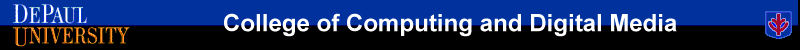﻿ CSC 478 - Programming Data Mining Applications - AssignmentsHome >> Assignments >> Assignment 1

# Assignment 1

## Due: Sunday, September 27

For this assignment you will experiment with Python. NumPy, and Pandas in order to perform some basic data preprocessing and analysis tasks.

You will work with a modified subset of a real data set of customer for a bank. The data is provided in a CSV formatted file with the first row containing the attribute names. The description of the the different fields in the data are provided in this document. You must only use Python, NumPy, Pandas, Matplotlib to perform the tasks for this assignment

1. Explore the general characteristics of the data as a whole: examine the means, standard deviations, and other statistics associated with the numerical attributes; also show the distributions of values associated with categorical attributes (for categorical attributes use Matplotlib library and/or plotting capabilities of Pandas to generate bar charts showing the distribution of categories for each attribute).
2. Suppose that the hypothetical bank is particularly interested in customers who buy the PEP (Personal Equity Plan) product. Compare and contrast the subsets of customers who buy and don't buy the PEP. Compute summaries (as in part 1) of the selected data with respect to all other attributes (you do not need to plot distributions). Can you observe any significant differences between these segments of customers? Discuss your observations.
3. Use z-score normalization to standardize the values of the income attribute. [Do not change the original income attribute or add the normalized income to the table.]
4. Discretize the age attribute into 3 categories (corresponding to "young", "mid-age", and "old"). [Do not change the original age attribute or add the discretized age to the table.]
5. Use Min-Max Normalization to transform the values of all numeric attributes  (income, age, children) in the original table onto the range 0.0-1.0.
6. Convert the table (after normalization in part 5) into the standard spreadsheet format. Note that this requires converting each categorical attribute into multiple binary ("dummy") attributes (one for each values of the categorical attribute) and assigning binary values corresponding to the presence or not presence of the attribute value in the original record). The numeric attributes should remain unchanged. Save this new table into a file called bank_numeric.csv and submit it along with your assignment.
7. Using the numeric data set with the dummy variables (of the previous part), perform basic correlation analysis among the attributes. Discuss your results by indicating any significant positive or negative correlations among pairs of attributes. You need to construct a complete Correlation Matrix. Be sure to first remove the Customer ID column before creating the correlation matrix. [Hint: you can create the correlation matrix by using the corr() function in Pandas or corrcoef function in NumPy].
8. Create a scatter plot of the (non-normalized) Income attribute relative to Age. Be sure that your plot contains appropriate labels for the axes. Do these variables seem correlated?
9. Create histograms for (non-normalized) Income (using 9 bins) and Age (using 15 bins).
10. Perform a cross-tabulation of the region attribute with the pep attribute. This requires the aggregation of the occurrences of each pep value (yes or no) separately for each value of the region attribute. Show the results as a 4 by 2 (region x pep) table with entries representing the counts. [Hint: you can either use Numpy or use aggregations functions in Pandas such as groupby() and  cross-tab().] Then, either using Matplotlib directly or the plot() function in Pandas create a bar chart graph to visualize of the relationships between these sets of variables. [Hint: This example of creating simple bar charts using Matplotlib may be useful.]
11. Now consider a modified version of the bank data that contains missing values (bank_data_missing_vals.csv). (a) Using Pandas determine all the attributes with missing values and the number of missing values for each such attribute. (b) Show all the instances in the data that contain a missing value. (c) Fill the missing values for all numeric attributes using the mean value for the attribute. (d) After filling in the missing numeric values, drop all rows where a categorical attribute contains a missing value. (e) Show that the final resulting table does not contain missing values.

Notes on Submission: You must submit your Jupyter Notebook (similar to examples in class) which includes your documented code, results of your interactions, and any discussions or explanations of the results. Please organize your notebook so that it's clear what parts of the notebook correspond to which problems in the assignment. Please submit the notebook in both IPYNB and HTML formats (along with any auxiliary files). Your assignment should be submitted via D2L.